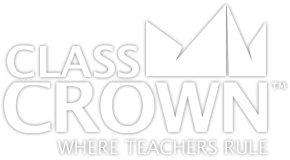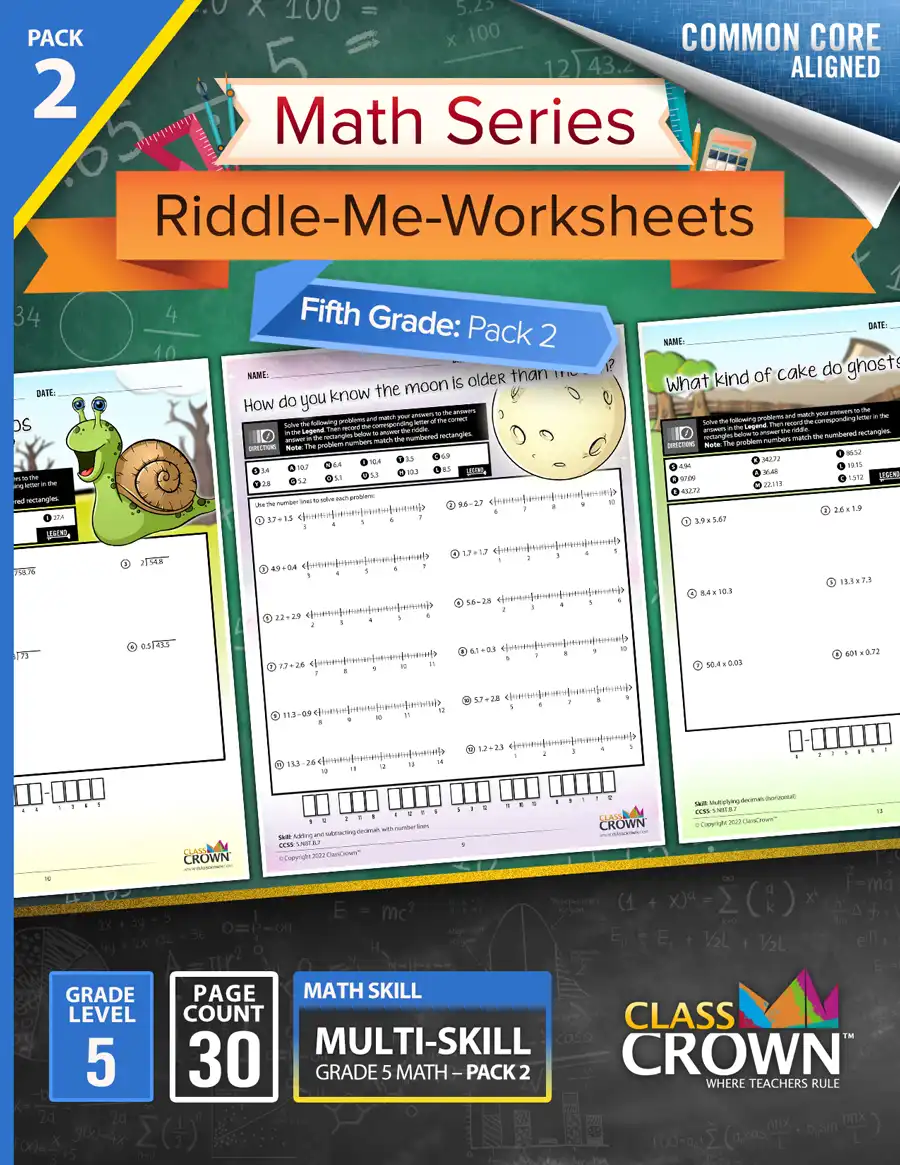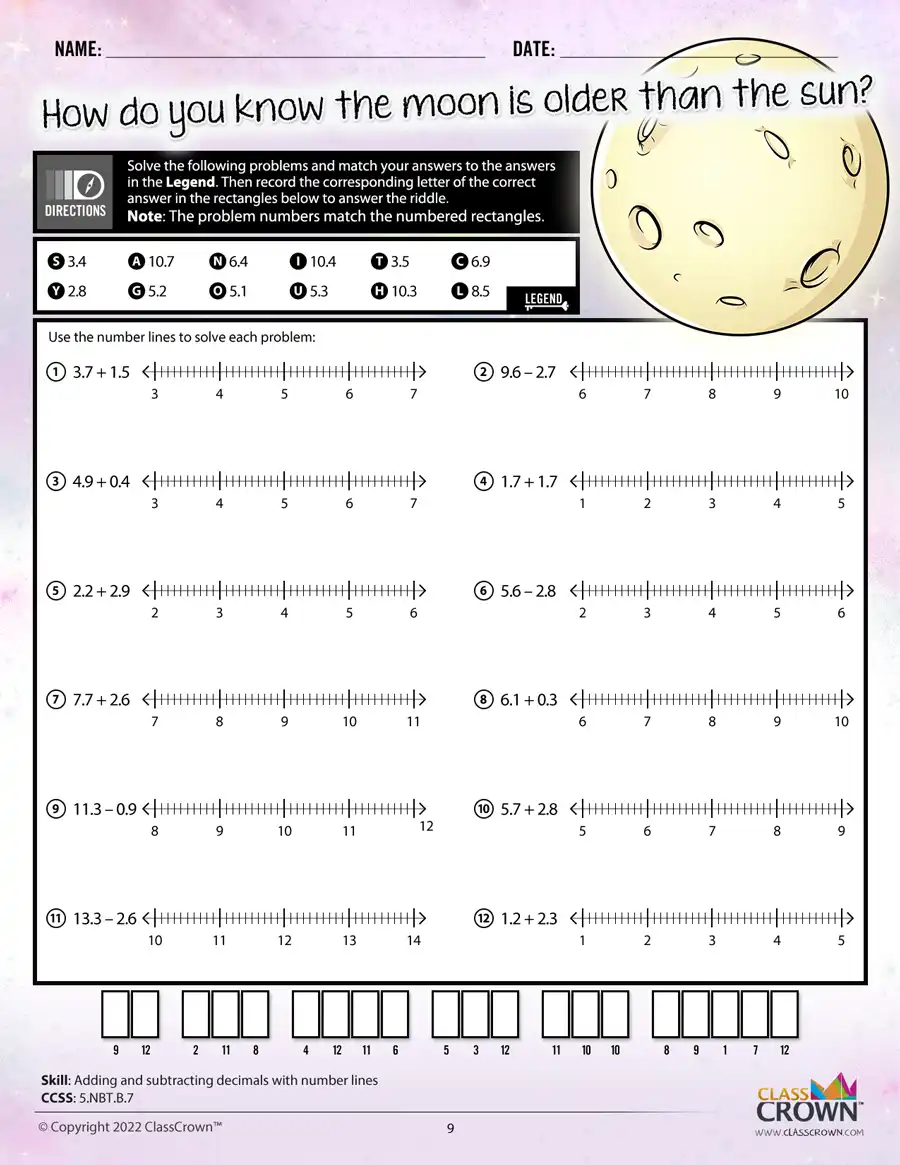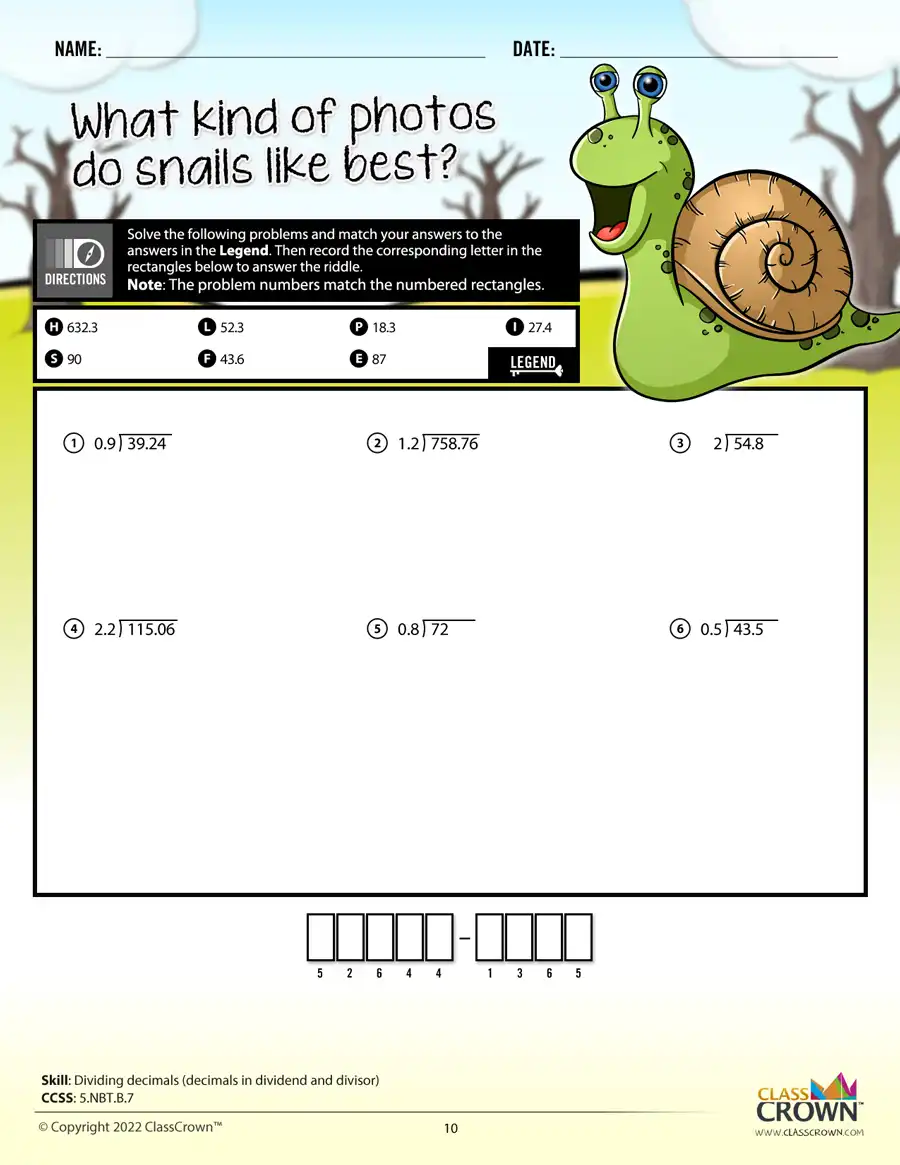Math Worksheets

# 5th Grade Math Worksheets: Pack 2

## Self-checking math worksheets with riddles to keep kids motivated.

Rating: 0/5
(0 Reviews)
5th
WORKSHEET COUNT
30
FORMAT(S)
PDF
Yes
\$8.99
The Details
Description
• Do your students ever complete an entire math worksheet, only to find that they have done most of the problems incorrectly and clearly have a misconception?
• ClassCrown’s Riddle-Me-Worksheets help to prevent this problem by introducing a puzzle aspect to math, giving students immediate feedback as to whether or not they are solving problems correctly. If the answer to the riddle isn't correct, the student knows which problems he's made an error on.
Specifics
• Subject:  Number lines, 4-digit Multiplication, plus more!
• Product Count:  30
• Full Page Count:  50
• File Format(s):  PDF

What's Included?
• Adding and subtracting decimals (horizontal)
• Adding and subtracting decimals word problems
• Adding and subtracting decimals with number lines
• Dividing decimals (decimals in dividend and divisor)
• Dividing decimals (decimals in dividend and divisor)
• Multiplying decimals (vertical)
• Multiplying decimals (horizontal)
• Multiplying decimals visually
• Multiplying decimals visually
• Adding and subtracting fractions with unlike denominators, visual
• Adding and subtracting fractions with unlike denominators
• Adding and subtracting mixed numbers with unlike denominators, no regrouping
• Adding and subtracting mixed numbers with unlike denominators, with regrouping
• Adding and subtracting fractions with unlike denominators, word problems
• Adding and subtracting fractions with unlike denominators and word problems review
• Fractions as division problems
• Examining fraction value
• Fractions as division problems, word problems
• Multiplying fractions by whole numbers with number lines
• Multiplying fractions, different denominators
• Multiplying mixed numbers
• Multiplying fractions, visual
• Finding and comparing products
• Estimating multiplication of fractions
• Multiplying fractions, word problems
• Multiplying fractions with cross-canceling
• Dividing fractions by whole numbers
• Dividing whole numbers by fractions
• Dividing whole numbers by fractions, visual
CCSS: 5.NBT.B.7, 5.NF.A.1, 5.NF.A.2, 5.NF.B.3, 5.NF.B.4A, 5.NF.B.4B, 5.NF.B.5A, 5.NF.B.5, 5.NF.B.6, 5.NF.B.7A, 5.NF.B.7B
Reviews
Overall Rating: 0/5
(Showing 0 of 0)
See More Reviews @ TpTSelf Checking

Our math worksheets introduce a puzzle aspect to math, giving students immediate feedback as to whether or not they are solving problems correctly. If the answer to the riddle isn't spelled correctly, the student knows which problems he's made an error on.Fun Puzzle AspectImmediate Feedback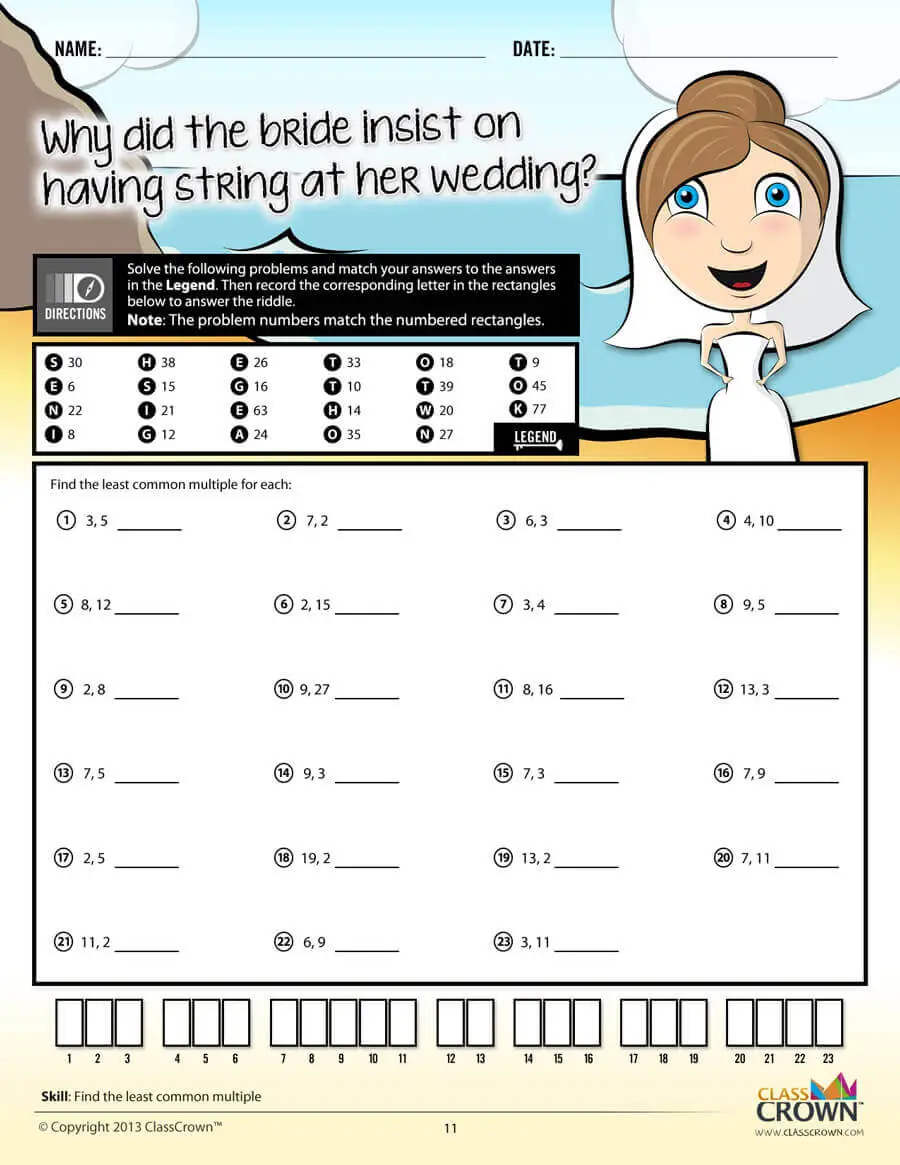Problem Solving Motivation

Each math worksheet contains a riddle that the student solves by completeing all the problems on the worksheet. This keeps kids motivated to complete each problem so that they can find the answer to the riddle.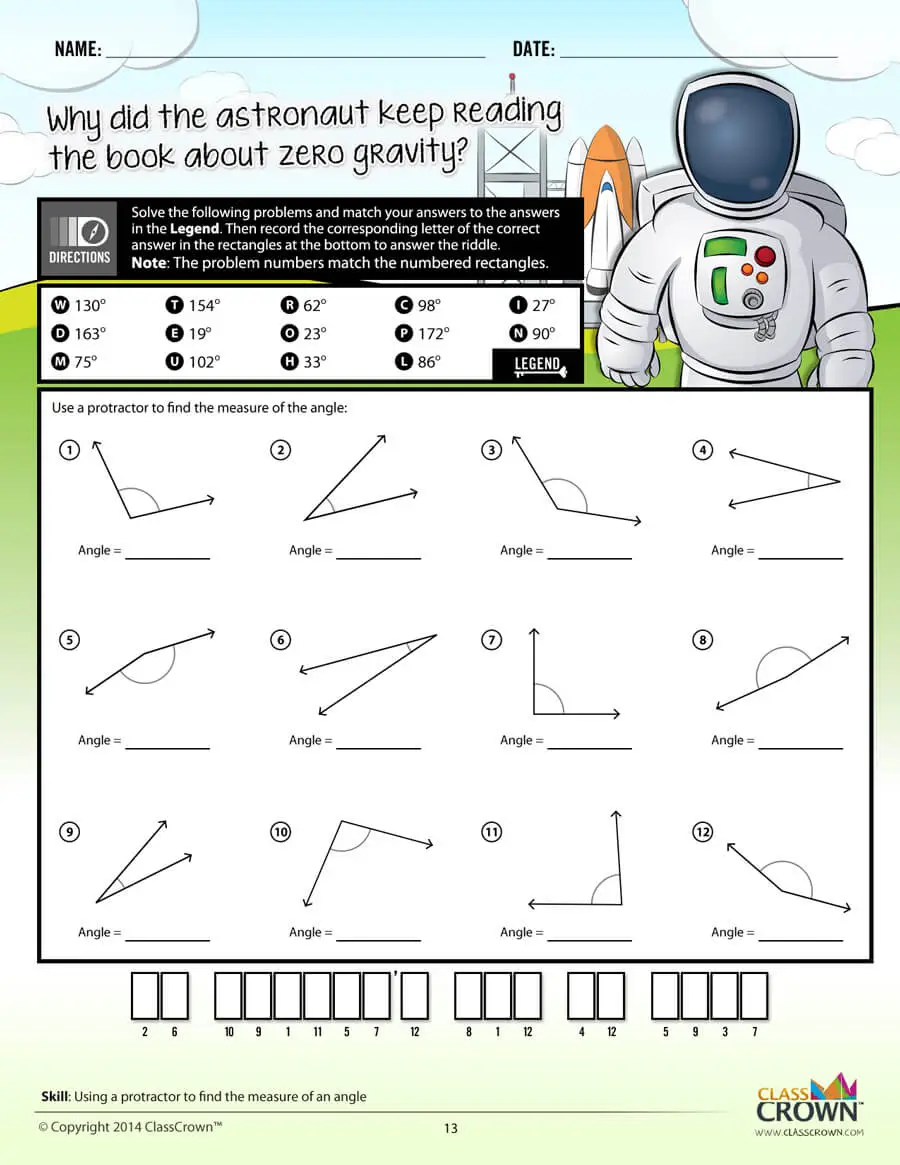Common Core Aligned

All our math worksheet packs are designed with Common Core in mind. That way you don’t have to worry about whether your math ciriculum is aligned or not when you incorpoate ClassCrown Riddle-Me-Worksheets in your lesson plans.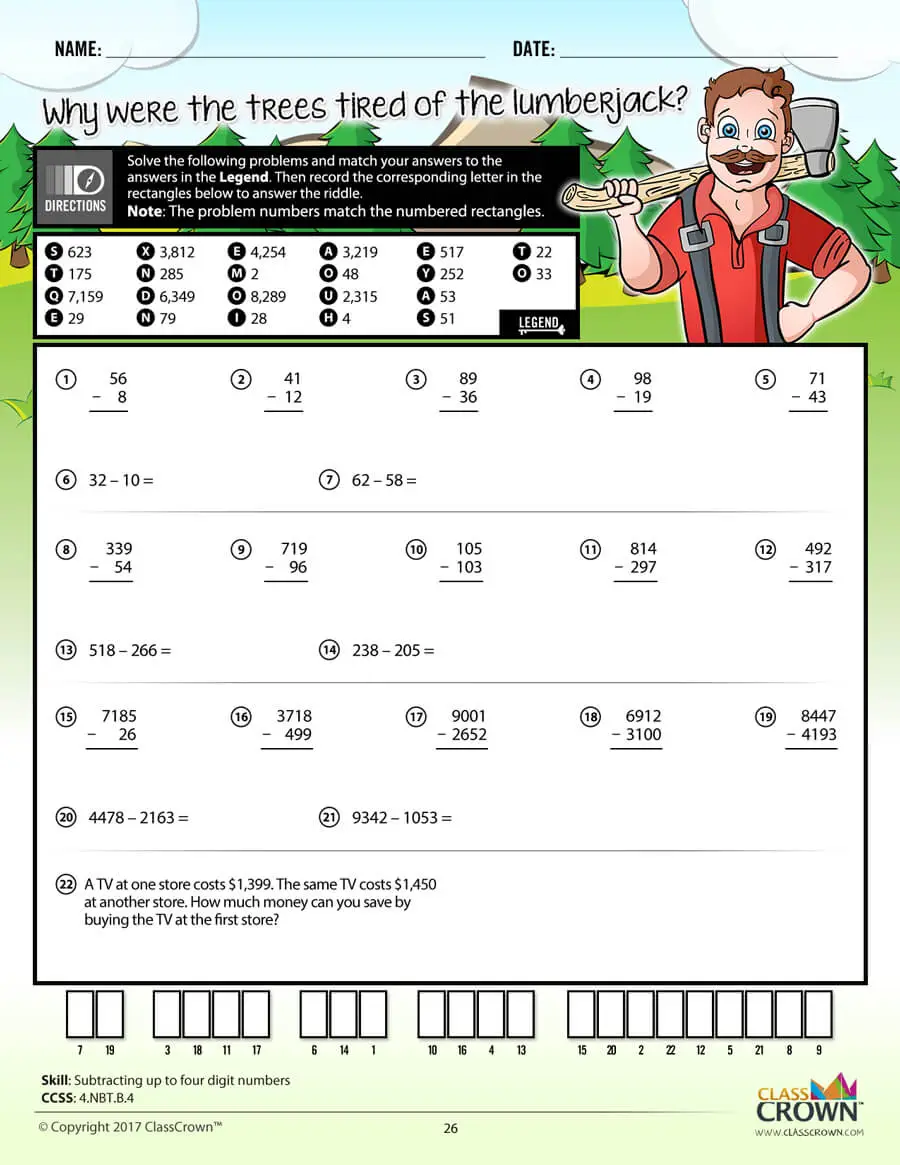High Quality Design

Each page of our math worksheets has been produced in high resolution at 144 dpi and designed in full, vibrant color for maximum quality. They look stunning whether you are printing in color or black and white.High Resolution
(144 dpi)Stunning Color & Clarity